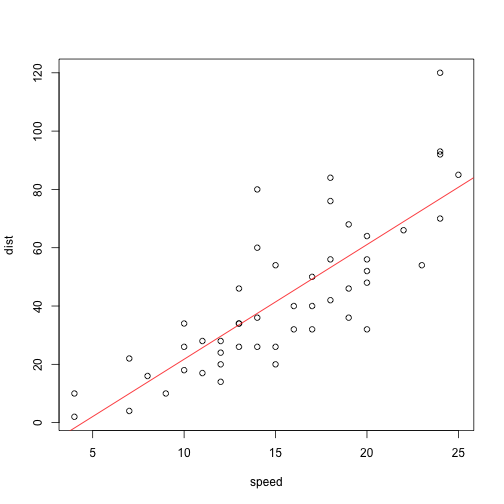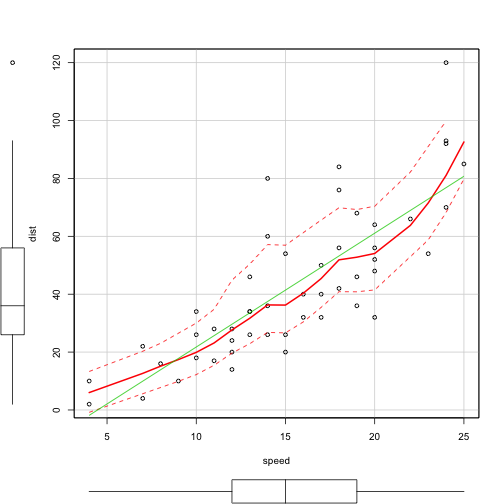Speed and stopping distance of cars

Introduction

The data give the speed of cars and the distances taken to stop. Note that the data were recorded in the 1920s.

library(car)
data(cars)
tail(cars)
##    speed dist
## 45    23   54
## 46    24   70
## 47    24   92
## 48    24   93
## 49    24  120
## 50    25   85

Results

We found that

out = lm(dist ~ speed, data = cars)
out
##
## Call:
## lm(formula = dist ~ speed, data = cars)
##
## Coefficients:
## (Intercept)        speed
##      -17.58         3.93
plot(cars)
abline(out, col = "red")scatterplot(dist ~ speed, data = cars)Conclusion

Stopping distance determined by square of vehicle speed.